Question

Anyone can solve these questions? (4) Let if (z, y)メ(0,0) if (x, y) (0,0) f(z, y)...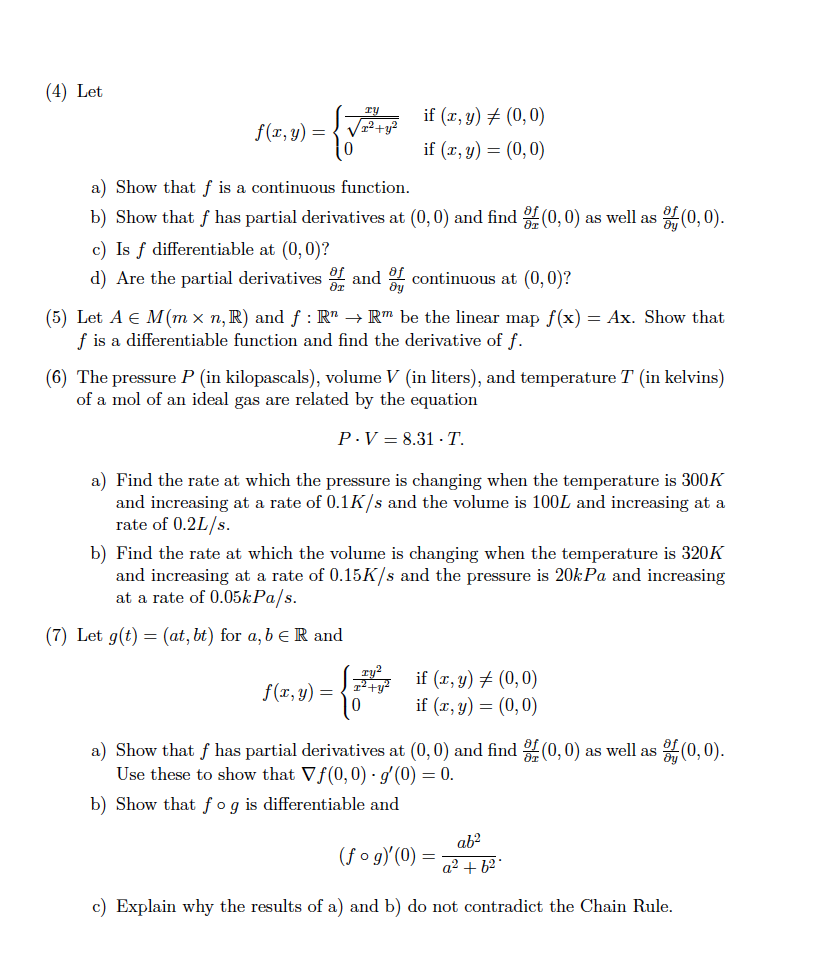Anyone can solve these questions?

(4) Let if (z, y)メ(0,0) if (x, y) (0,0) f(z, y) / 0 a) Show that f is a continuous function b) Show that f has partial derivatives at (0,0) and find (0,0) as well as c) Is f differentiable at (0, 0)? d) Are the partial derivatives r tinuous at (0,0)? (0,0) (5) Let A E M(mx n,R) and f : RnRm be the linear map f(x)-Ax. Show that f is a differentiable function and find the derivative of f. (6) The pressure P (in kilopascals), volume V (in liters), and temperature T (in kelvins) of a mol of an ideal gas are related by the equation P. V-8.31 . T. a) Find the rate at which the pressure is changing when the temperature is 300K and increasing at a rate of 0.1K/s and the volume is 100L and increasing at a rate of 0.2L/s b) Find the rate at which the volume is changing when the temperature is 320K and increasing at a rate of 0.15K/s and the pressure is 20kPa and increasing at a rate of 0.05kPa/s (7) Let g(t) = (at, bt) for a,be R and if(x, y) if (x, y) (0,0) (0,0) f(x, y) 0 a) Show that f has partial derivatives at (0,0) and find 쓿(0,0) as well as (0,0) Use these to show that ▽f(0,0) . g'(0) b) Show that f og is differentiable and 0 c) Explain why the results of a) and b) do not contradict the Chain Rule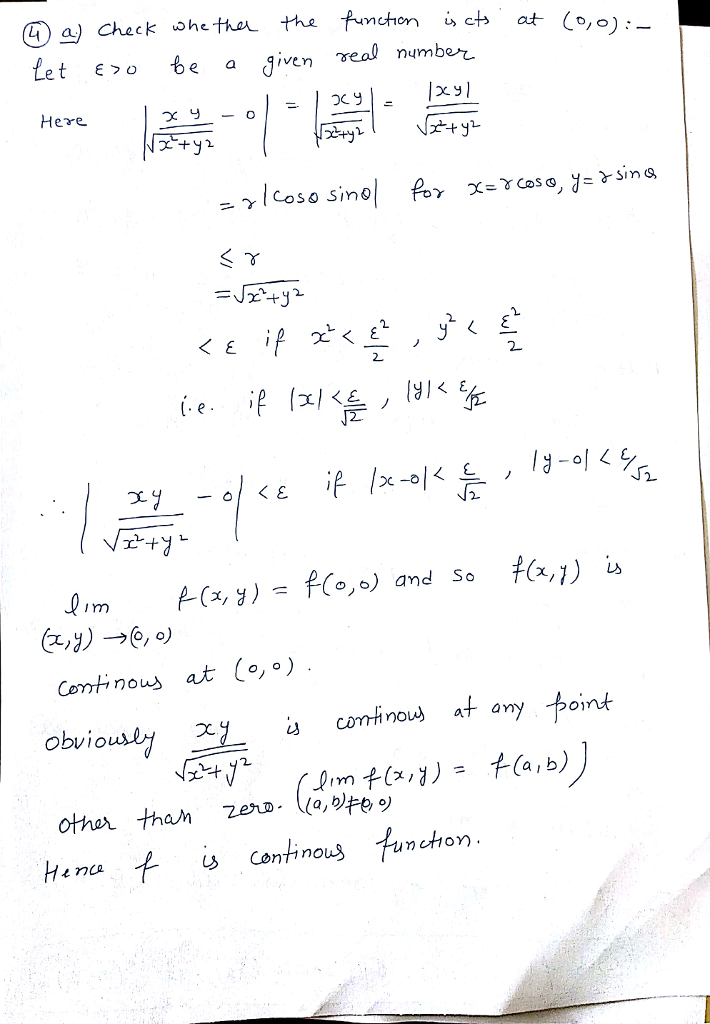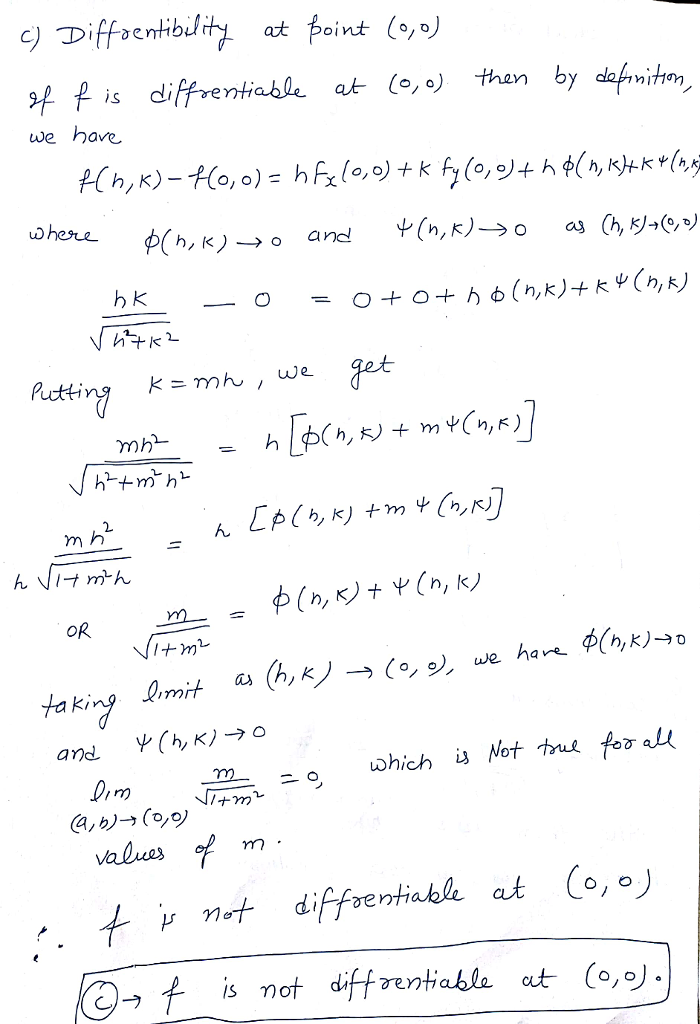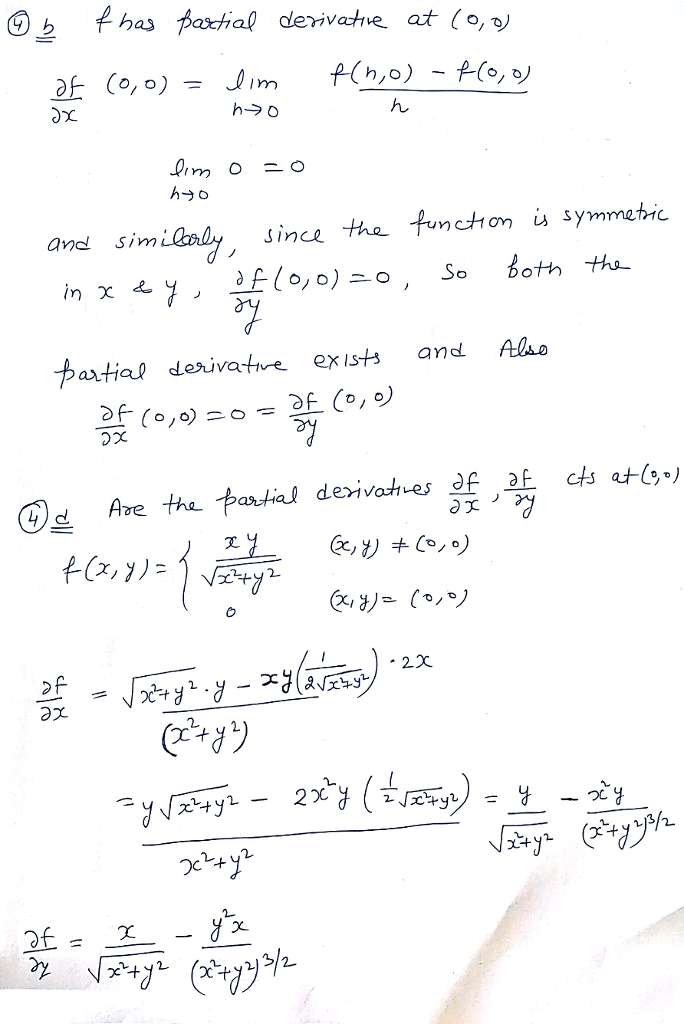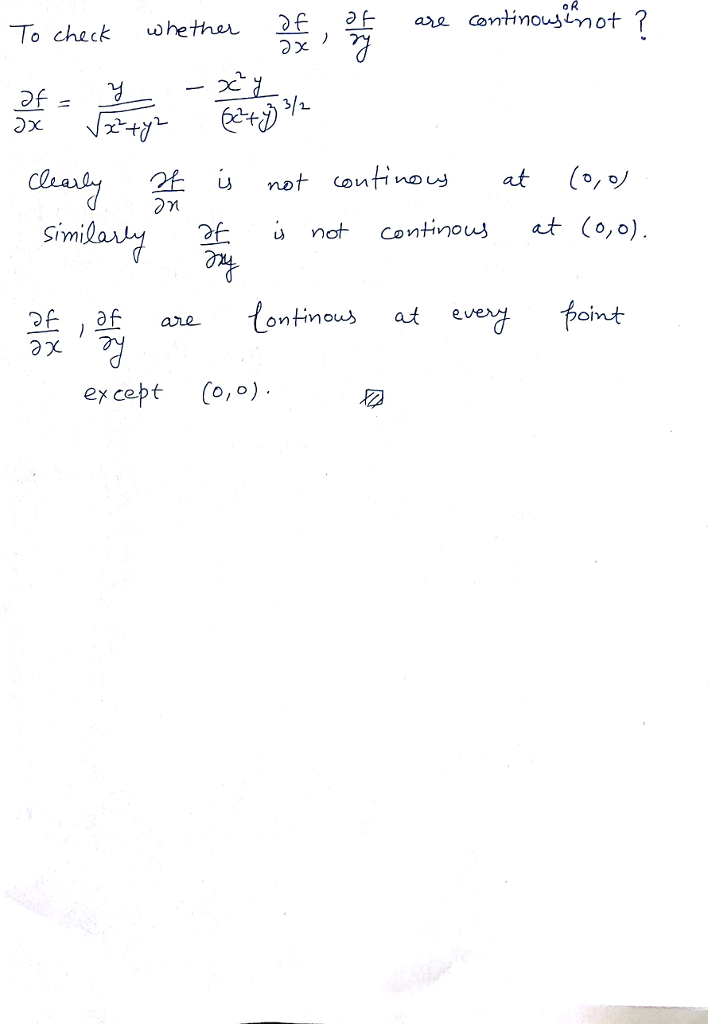Earn Coins

Coins can be redeemed for fabulous gifts.

Similar Homework Help Questions
• *Let f : R2 -R be given by z, y)(0,0 r, y)- 2y and f(0,0) =...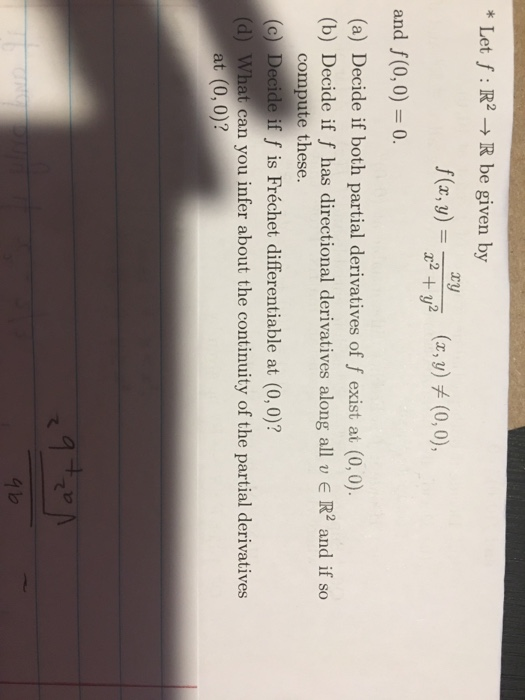*Let f : R2 -R be given by z, y)(0,0 r, y)- 2y and f(0,0) = 0. (a) Decide if both partial derivatives of f exist at (0, 0) (b) Decide if f has directional derivatives along all v R2 and if so compute these. (c) Decide if f is Fréchet differentiable at (0, 0)? (d) What can you infer about the continuity of the partial derivatives at (0, 0)? て

• Exercice 2 (5pts) Let f given by f(x, y) Isinyif (x, y) (0,0) and f(0,0) 0...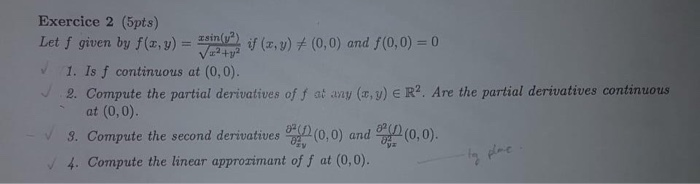Exercice 2 (5pts) Let f given by f(x, y) Isinyif (x, y) (0,0) and f(0,0) 0 1V224 1. Is f continuous at (0,0). 2. Compute the partial derivatives of f at any (x, y) E R2. Are the partial derivatives continuous (0,0). at (0,0) (0,0) and 3. Compute the second derivatives 4. Compute the linear approzimant of f at (0,0). Exercice 2 (5pts) Let f given by f(x, y) Isinyif (x, y) (0,0) and f(0,0) 0 1V224 1. Is f...

• 2. Consider the function f : R2 → R defined below. r3уг_ if (x,y) (0,0) f(x,y)...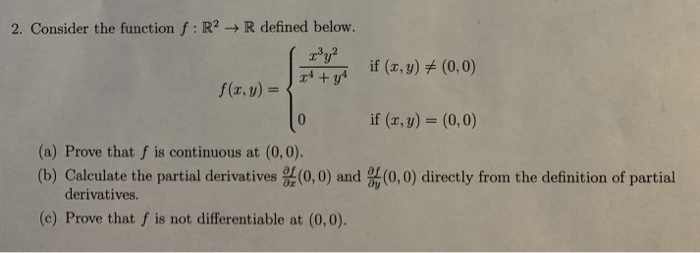2. Consider the function f : R2 → R defined below. r3уг_ if (x,y) (0,0) f(x,y) = if (x, y) (0, 0) (a) Prove that f is continuous at (0,0) (b) Calculate the partial derivatives (0,0) and (0,0) directly from the definition of partial derivatives. (c) Prove that f is not differentiable at (0,0).

• Question 2 (20 points): Consider the functions f(x, y)-xe y sin y and g(x, y)-ys 1. Show f is differentiable in its domain 2. Compute the partial derivatives of g at (0,0) 3. Show that g is not d...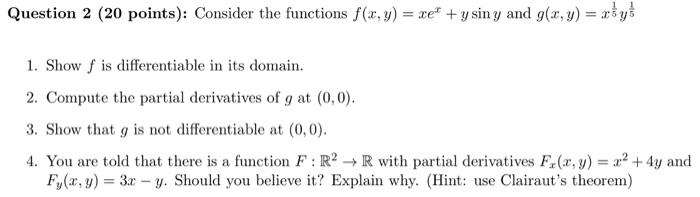Question 2 (20 points): Consider the functions f(x, y)-xe y sin y and g(x, y)-ys 1. Show f is differentiable in its domain 2. Compute the partial derivatives of g at (0,0) 3. Show that g is not differentiable at (0,0) 4. You are told that there is a function F : R2 → R with partial derivatives F(x,y) = x2 +4y and Fy(x, y 3x - y. Should you believe it? Explain why. (Hint: use Clairaut's theorem) Question 2...

• = f ху Consider the function f(x,y) if x² + y² (x, y) + (0,0) =...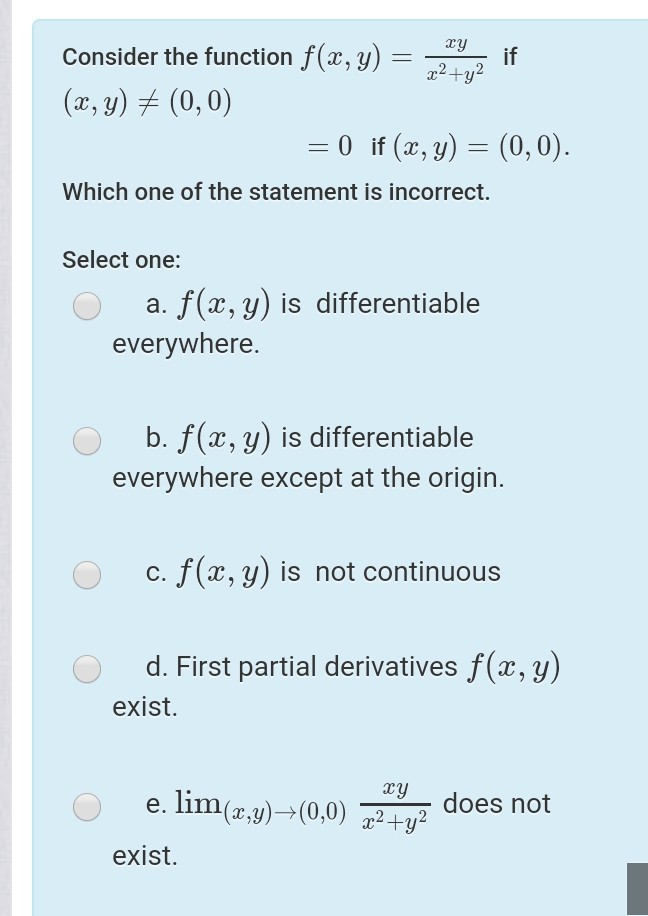= f ху Consider the function f(x,y) if x² + y² (x, y) + (0,0) = 0 if (x, y) = (0,0). Which one of the statement is incorrect. Select one: a. f(x,y) is differentiable everywhere. b. f(x,y) is differentiable everywhere except at the origin. c. f(x,y) is not continuous d. First partial derivatives f(x,y) exist. ху e. lim(x,y)—(0,0) x2 + y2 does not exist.

• b) i. Using e-8 definition show that f is continuous at (0,0), where f(x,y) = {aš...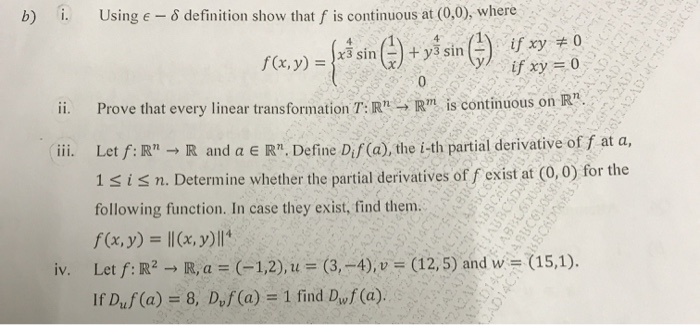b) i. Using e-8 definition show that f is continuous at (0,0), where f(x,y) = {aš sin () + yś sin () if xy + 0 242ADES if xy = 0 ii. Prove that every linear transformation T:R" - R" is continuous on R". iii. Let f:R" → R and a ER" Define Dis (a), the i-th partial derivative of f at a, 1 sisn. Determine whether the partial derivatives of f exist at (0,0) for the following function. In...

• if (r.y) (0,0), 0,f (, y) (0, 0) 2. Consider f : IR2 -R defined by f(r,y)-+ (a) Show by explicit computation that the directional derivative exists at (x, y)- (0,0) for all oi rections u є R2 with 1...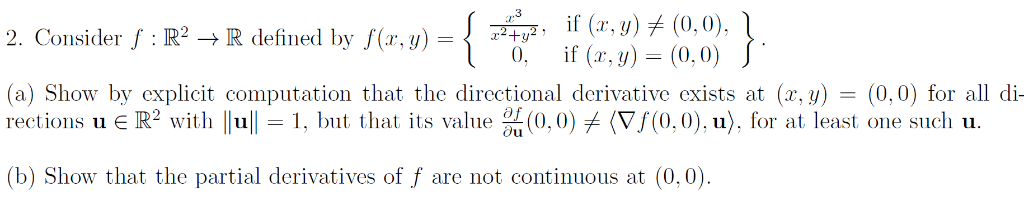if (r.y) (0,0), 0,f (, y) (0, 0) 2. Consider f : IR2 -R defined by f(r,y)-+ (a) Show by explicit computation that the directional derivative exists at (x, y)- (0,0) for all oi rections u є R2 with 1 11-1, but that its value %(0.0) (Vf(0,0).u), fr at least one sucli u. (b) Show that the partial derivatives of f are not continuous at (0,0) if (r.y) (0,0), 0,f (, y) (0, 0) 2. Consider f : IR2 -R...

• Problem 3. Define the function: 2+_ 0 if (z,y)#10.0) if (a,y)-(0,0) f(x, v)= (a) Graph the...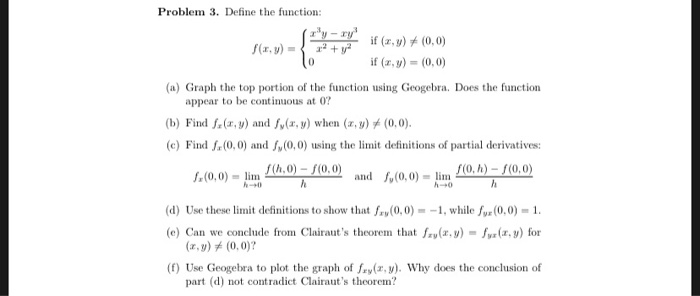Problem 3. Define the function: 2+_ 0 if (z,y)#10.0) if (a,y)-(0,0) f(x, v)= (a) Graph the top portion of the function using Geogebra. Does the function appear to be continuus at 0? (b) Find fz(z, y) and fy(z, y) when (z, y) #10.0) (c) Find f(0,0) and s,(0,0) using the limit definitions of partial derivatives and f,(0,0)-lim rah) - f(O,0) d) Use these limit definitions to show that fay(0,0)--1, while x(0,0)-1 (e) Can we conclude from Clairaut's theorem that()-yr(x,y) for...

• Let f(x,y) = (x" + 2?y?)!. compute all second-order partial derivatives of fat (0,0), if they...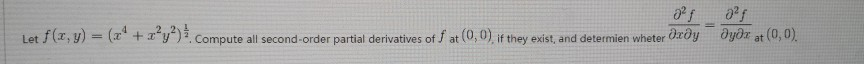Let f(x,y) = (x" + 2?y?)!. compute all second-order partial derivatives of fat (0,0), if they exist, and determien wheter dæðyəyər at (0,0).

• Consider the function f: RR given by if (x, y)メ(0,0) íf (z, y) = (0,0). f(z,...Consider the function f: RR given by if (x, y)メ(0,0) íf (z, y) = (0,0). f(z, y)=(0+r R2 does the directional derivative Duf(0,0) exist? Evaluate the For which vectors 0メu directional derivative wherever it exists. 4 marks